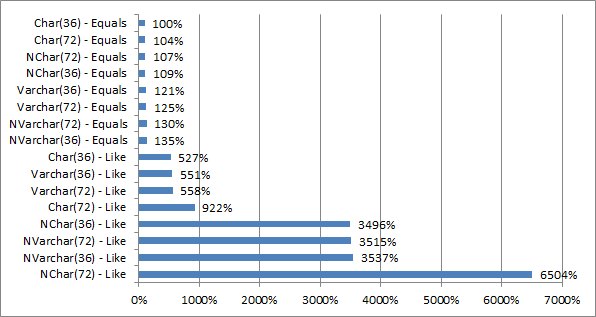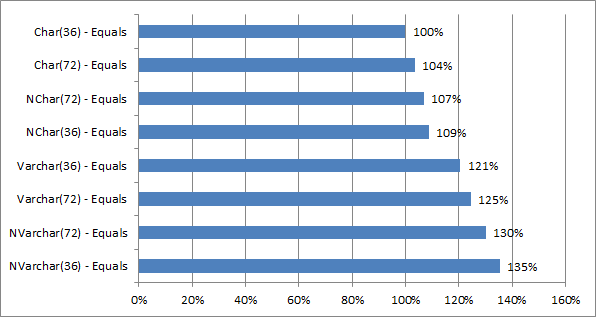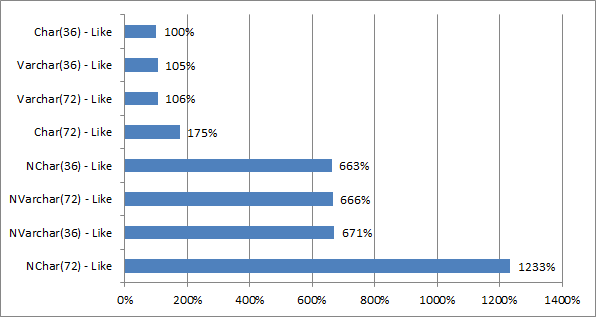Eli Weinstock-Herman

# Performance Impacts of Unicode, Equals vs LIKE, and Partially Filled Fixed Width

Original post posted on December 28, 2011 at LessThanDot.com

Several weeks ago I was refreshing my memory on some nvarchar/varchar tradeoffs when I ran into a post by Michael J Swart (blog|twitter) where he shared the results of investigating a performance problem in one of his live environments. After changing many columns from varchar to nvarchar, he had several procedures that were taking much longer to execute without any changes in I/O profile. This led him to compare the execution times of searching a varchar vs an nvarchar column, where he found that searching an nvarchar took 800% as long as a varchar.

After reading this, I was curious. Does that follow with char and nchar also? How about looking for exact matches (equals) instead of a search (LIKE)? And partially filled char fields are supposed to be poorer performing too, right?

## Results

The results are based on 4 runs of each query against 1,000,000 rows with explicitly limited parallelism. The name identifies the type, which operation was used (equals or LIKE), and the size of the field. All data was 36 characters, so "(36)" tests are fully populated values and "(72)" tests are half-full values.All Results, Normalized to Shortest Value (Char 32 - Equals)

This graph shows the average for each type as a percentage of the smallest value, 'Char(72) - Equals'. What's immediately obvious is the level of difference between an equals operation and a LIKE, especially when we get to the trailing, partially filed NCHAR field (wow!).

The data was pretty consistent across the 4 runs used for these graphs:

TestAverage (ms)StdDev (ms)
Char(36) - Equals1130
Char(36) - Like5960
Char(72) - Equals1172
Char(72) - Like10431.5
NChar(36) - Equals1230
NChar(36) - Like395113.08
NChar(72) - Equals1211.5
NChar(72) - Like73500
NVarchar(36) - Equals1530
NVarchar(36) - Like39978.85
NVarchar(72) - Equals1472
NVarchar(72) - Like39723
Varchar(36) - Equals1362.87
Varchar(36) - Like6232.45
Varchar(72) - Equals1411.5
Varchar(72) - Like6300

Note: I originally had 5 runs, but removed the first since it had consistently higher values, with 2 cases far outside several standard devitations.

### Comparing EQUALS statements

There is limited statistical significance in the fixed-width EQUALs, but the values do group together by type.Equals Results, Normalized to Shortest Value (Char 32 - Equals)

It is interesting to note that there is a pretty consistent 20% gap between fixed and variable widths for the same types (char to varchar, for instance), regardless of whether the column is partially or fully populated.

### Comparing LIKE statements

There is a much broader impact when we start looking at the LIKE statement comparisons. Like Michael's post above, there is a noticeable difference (on my system, ~650%) between searching a varchar and an nvarchar column.LIKE Results, Normalized to Shortest Value (Char 32 - Like)

The conclusions I see are:

• The previously mentioned difference between unicode and non-unicode fields is present
• Fixed width fields suffer the same performance impact as variable length
• Partially populated fixed width fields ran 75-90% longer than the associated fully populated column
• Unicode fields were consistently 6x slower (on my system) than their non-unicode variants

Poorly sized nchar seems to be the real winner.

## Scripts

The script for these results was heavily based on the one in Michael's post above. The prep script assumes you have a numbers table (here is a script for one).

Setup Sample Data Table

Code: tsql
-----------------------------------
-- Prep - Generate Sample Data

IF EXISTS (SELECT 1 FROM INFORMATION_SCHEMA.TABLES WHERE TABLE_SCHEMA = 'dbo' AND TABLE_NAME = 'CharTypeTest')
DROP TABLE dbo.CharTypeTest;

CREATE TABLE dbo.CharTypeTest(
CharTypeTestId int identity(1,1) NOT NULL,
VarcharField36 varchar(36) NOT NULL,
VarcharField72 varchar(72) NOT NULL,
CharField36 char(36) NOT NULL,
CharField72 char(72) NOT NULL,
NVarcharField36 nvarchar(36) NOT NULL,
NVarcharField72 nvarchar(72) NOT NULL,
NCharField36 nchar(36) NOT NULL,
NCharField72 nchar(72) NOT NULL );

WITH SampleData AS(
SELECT CAST(NEWID() as CHAR(36)) AS SampleText
FROM Numbers N1, Numbers N2           -- 50,000 * 20 = 1,000,000
WHERE N1.Number < 50000 AND N2.Number < 20
)

INSERT INTO dbo.CharTypeTest(VarcharField36, CharField36, NvarcharField36, NCharField36,
VarcharField72, CharField72, NvarcharField72, NCharField72)
SELECT SampleText, SampleText, SampleText, SampleText, SampleText, SampleText, SampleText, SampleText
FROM SampleData;

And here is the test code:

Setup Sample Data Table

Code: tsql
-----------------------------
-- Test

CREATE TABLE #Results (name varchar(30), elapsed int, run int);
DECLARE @StartTime DateTime, @run int;
SELECT @run = 1;

WHILE (SELECT COUNT(1) FROM #Results WHERE name = 'Char(36) - Equals') < 2
BEGIN

SET @StartTime = GETDATE();
SELECT COUNT(1) FROM CharTypeTest WHERE CharField36 = 'abcd' OPTION (MAXDOP 1);
INSERT INTO #Results(name,elapsed,run) VALUES('Char(36) - Equals', DateDiff(ms, @StartTime, GETDATE()),@run);

SET @StartTime = GETDATE();
SELECT COUNT(1) FROM CharTypeTest WHERE VarcharField36 = 'abcd' OPTION (MAXDOP 1);
INSERT INTO #Results(name,elapsed,run) VALUES('Varchar(36) - Equals', DateDiff(ms, @StartTime, GETDATE()),@run);

SET @StartTime = GETDATE();
SELECT COUNT(1) FROM CharTypeTest WHERE NCharField36 = N'abcd' OPTION (MAXDOP 1);
INSERT INTO #Results(name,elapsed,run) VALUES('NChar(36) - Equals', DateDiff(ms, @StartTime, GETDATE()),@run);

SET @StartTime = GETDATE();
SELECT COUNT(1) FROM CharTypeTest WHERE NVarcharField36 = N'abcd' OPTION (MAXDOP 1);
INSERT INTO #Results(name,elapsed,run) VALUES('NVarchar(36) - Equals', DateDiff(ms, @StartTime, GETDATE()),@run);

SET @StartTime = GETDATE();
SELECT COUNT(1) FROM CharTypeTest WHERE CharField36 LIKE '%abcd%' OPTION (MAXDOP 1);
INSERT INTO #Results(name,elapsed,run) VALUES('Char(36) - Like', DateDiff(ms, @StartTime, GETDATE()),@run);

SET @StartTime = GETDATE();
SELECT COUNT(1) FROM CharTypeTest WHERE VarcharField36 LIKE '%abcd%' OPTION (MAXDOP 1);
INSERT INTO #Results(name,elapsed,run) VALUES('Varchar(36) - Like', DateDiff(ms, @StartTime, GETDATE()),@run);

SET @StartTime = GETDATE();
SELECT COUNT(1) FROM CharTypeTest WHERE NCharField36 LIKE N'%abcd%' OPTION (MAXDOP 1);
INSERT INTO #Results(name,elapsed,run) VALUES('NChar(36) - Like', DateDiff(ms, @StartTime, GETDATE()),@run);

SET @StartTime = GETDATE();
SELECT COUNT(1) FROM CharTypeTest WHERE NVarcharField36 LIKE N'%abcd%' OPTION (MAXDOP 1);
INSERT INTO #Results(name,elapsed,run) VALUES('NVarchar(36) - Like', DateDiff(ms, @StartTime, GETDATE()),@run);

SET @StartTime = GETDATE();
SELECT COUNT(1) FROM CharTypeTest WHERE CharField72 = 'abcd' OPTION (MAXDOP 1);
INSERT INTO #Results(name,elapsed,run) VALUES('Char(72) - Equals', DateDiff(ms, @StartTime, GETDATE()),@run);

SET @StartTime = GETDATE();
SELECT COUNT(1) FROM CharTypeTest WHERE VarcharField72 = 'abcd' OPTION (MAXDOP 1);
INSERT INTO #Results(name,elapsed,run) VALUES('Varchar(72) - Equals', DateDiff(ms, @StartTime, GETDATE()),@run);

SET @StartTime = GETDATE();
SELECT COUNT(1) FROM CharTypeTest WHERE NCharField72 = N'abcd' OPTION (MAXDOP 1);
INSERT INTO #Results(name,elapsed,run) VALUES('NChar(72) - Equals', DateDiff(ms, @StartTime, GETDATE()),@run);

SET @StartTime = GETDATE();
SELECT COUNT(1) FROM CharTypeTest WHERE NVarcharField72 = N'abcd' OPTION (MAXDOP 1);
INSERT INTO #Results(name,elapsed,run) VALUES('NVarchar(72) - Equals', DateDiff(ms, @StartTime, GETDATE()),@run);

SET @StartTime = GETDATE();
SELECT COUNT(1) FROM CharTypeTest WHERE CharField72 LIKE '%abcd%' OPTION (MAXDOP 1);
INSERT INTO #Results(name,elapsed,run) VALUES('Char(72) - Like', DateDiff(ms, @StartTime, GETDATE()),@run);

SET @StartTime = GETDATE();
SELECT COUNT(1) FROM CharTypeTest WHERE VarcharField72 LIKE '%abcd%' OPTION (MAXDOP 1);
INSERT INTO #Results(name,elapsed,run) VALUES('Varchar(72) - Like', DateDiff(ms, @StartTime, GETDATE()),@run);

SET @StartTime = GETDATE();
SELECT COUNT(1) FROM CharTypeTest WHERE NCharField72 LIKE N'%abcd%' OPTION (MAXDOP 1);
INSERT INTO #Results(name,elapsed,run) VALUES('NChar(72) - Like', DateDiff(ms, @StartTime, GETDATE()),@run);

SET @StartTime = GETDATE();
SELECT COUNT(1) FROM CharTypeTest WHERE NVarcharField72 LIKE N'%abcd%' OPTION (MAXDOP 1);
INSERT INTO #Results(name,elapsed,run) VALUES('NVarchar(72) - Like', DateDiff(ms, @StartTime, GETDATE()),@run);

SELECT @run = @run + 1;
END;

SELECT * FROM #Results;

DROP TABLE #Results;

I realize this is not a groundbreaking post, but after finding the results out for myself I thought it would be interesting to share. It also underlines and italicizes the need to fit your data definitions to the data you will be storing.

Comments are available on the original post at lessthandot.com